# Nyquist Rate and Antialiasing Filter Explained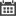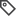Software & Firmware Design

In mud pulse telemetry, we use a sync signal for synchronization. A commonly used sync signal are four equally spaced pulses. The sync signal allows us to align the data properly for decoding. After synchronization, pulse locations are used to decode. If the digital signal we are analyzing is corrupted with missing or false pulses, the distorted signal can lead to missing syncs and false decodes. One possibly source of distortion is aliasing.

Aliasing happens anytime we sample a signal. Aliasing is an effect that causes different signals to look the same once sampled. This arises when we do not satisfy the Nyquist rate, which stems from the Nyquist Theorem. The Nyquist Theorem states that our sample rate must be at least twice the highest frequency component in our signal for an accurate representation. This minimum sample rate is known as the Nyquist rate. A simple example of aliasing, where two different sinusoids look the same after sampling, is shown in Figure 1. Since any signal can be represented as or is equivalent to a sum of sinusoids, this aliasing effect can cause a lot of distortion or misinformation in our digital signal. To help reduce the artifacts caused by aliasing, an antialiasing or lowpass filter is typically applied to limit the bandwidth of our signal.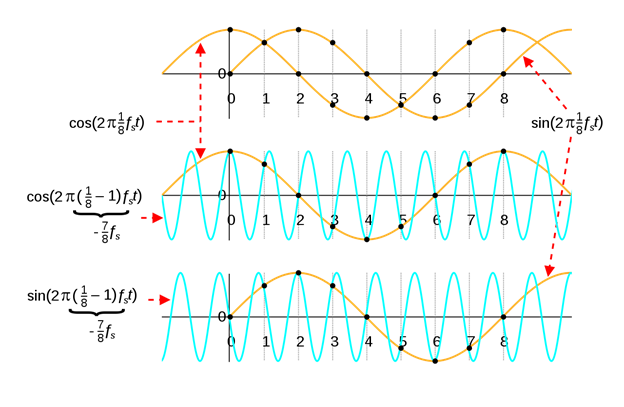Aliasing is a result of the Convolution Theorem, which states that convolution in time domain corresponds to multiplication in the frequency domain (1) and vice versa (2).

F[x(t)*y(t)]=X(jω) Y(jω) (1)
F[x(t) y(t)]=X(jω)*Y(jω) (2)

When we sample a signal x(t), we are essentially multiplying our signal by an impulse train p(t), where T is our sampling interval and f_s=1/T is our sampling frequency.

z[n]=x(nT)=x(t) p(t) (3)
p(t)=∑_(k=-∞)^∞▒〖δ(t-kT)〗 (4)

This multiplication results in the convolution of their Fourier transforms in the frequency domain. However, the Fourier transform of an impulse train is just an impulse train with a period equal to our sampling rate f_s. For illustration purposes, let us say x(t) is bandlimited with the highest frequency component of B.
This results in the Fourier transform of our sampled signal to be a sum of multiple copies of X(f) shifted by 〖nf〗_s shown in Figure 2 and 3. From this simple, illustration you can see that if B is greater than the Nyquist rate, f_s/2 the copies would overlap causing aliasing.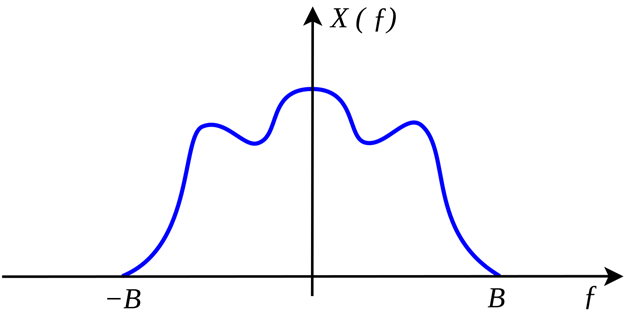Figure 2. Fourier Transform of our example signal. https://upload.wikimedia.org/wikipedia/commons/thumb/f/f7/Bandlimited.svg/1280px-Bandlimited.svg.png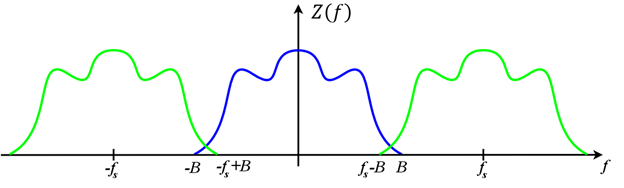Figure 3. Fourier Transform of our sampled signal. https://upload.wikimedia.org/wikipedia/commons/d/d5/AliasedSpectrum.png

### Recent Posts:Linear phase and computation/memory complexity are important characteristics to [..]Recap In my previous blog, we took a look at how to implement OAuth2 in LabVIEW. [..]3D Printing and its widespread use has been a long time coming and seems to have [..]Assumptions: Machine has a Nvidia CUDA Core GPU (such as a GeForce) with installed [..]Learning new skills can be quite the task especially when there's already so much [..]You are out with your friends, bird watching, and nothing could be more peaceful. [..]When analyzing data from multiple sources, we often find that the time series data [..]